ExerArduinoCompElet

Ardublock is a graphical programming environment to make programming physical computing with Arduino as easy as drag and drop.

http://blog.ardublock.com/

 Exercícios Arduino Arduino via Internet 2 CArlosP Arduino Via bluetooth Controlar Motor Step Arduino Motores reaproveitados de impressoras ou ...

 LOOP Exemplo completo IR (remote Control) Exemplo LCD serial I2C Completo Controlar Arduino via Internet 3 led Ardudroid

topo

Exercícios Arduino Componentes Eletrónicos

ExerArduinoCompElet

Examples > Digital I/O

LED – mais curto é o negativo

ArduBlocks requisitos de instalação

C:\...\...\Documents\Arduino\tools\ArduBlockTool\tool

Criar a estrutura de pastas acima e colar o ficheiro do ardublocks dentro da pasta tools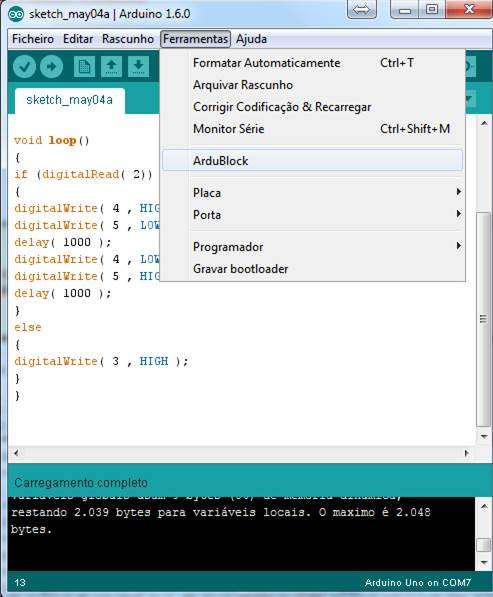9

In most programming languages, the first program you write prints "hello world" to the screen. Since an Arduino board doesn't have a screen, we blink an LED instead.

The boards are designed to make it easy to blink an LED using digital pin 13. Some like the Diecimila and LilyPad) have the LED built-in to the board. On most others (like the Mini and BT), there is a 1 KB resistor on the pin, allowing you to connect an LED directly. (To connect an LED to another digital pin, you should use an external resistor.) LEDs have polarity, which means they will only light up if you orient the legs properly. The long leg is typically positive, and should connect to pin 13. The short leg connects to GND; the bulb of the LED will also typically have a flat edge on this side. If the LED doesn't light up, trying reversing the legs (you won't hurt the LED if you plug it in backwards for a short period of time).

Na maioria das linguagens de programação, o primeiro programa escreve impressões "Olá mundo" para o ecrã. Desde que placa Arduino não tem um ecrã, vamos piscar um LED em vez disso.

As placas são projetadas para tornar mais fácil para piscar um LED usando pino digital 13.

A perna + curta conecta ao GND ; a lâmpada do LED será também tipicamente tem uma borda plana sobre este lado .

FILE > Examples > 01. Basics > Blink

The example code is very simple, credits are to be found in the comments.

* ------------

*

* turns on and off a light emitting diode(LED) connected to a digital

* pin, in intervals of 2 seconds. Ideally we use pin 13 on the Arduino

* board because it has a resistor attached to it, needing only an LED

*

* Created 1 June 2005

* copyleft 2005 DojoDave <http://www.0j0.org>

* http://arduino.berlios.de

*

* based on an orginal by H. Barragan for the Wiring i/o board

*/

int ledPin = 13; // LED connected to digital pin 13

void setup()

{

pinMode(ledPin, OUTPUT); // sets the digital pin as output

}

void loop()

{

digitalWrite(ledPin, HIGH); // sets the LED on

delay(1000); // waits for a second

digitalWrite(ledPin, LOW); // sets the LED off

delay(1000); // waits for a second

}

Sometimes you need to blink an LED (or some other time sensitive function) at the same time as something else (like watching for a button press). That means you can't use delay(), or you'd stop everything else the program while the LED blinked. Here's some code that demonstrates how to blink the LED without using delay(). It keeps track of the last time it turned the LED on or off. Then, each time through loop() it checks if a sufficient interval has passed - if it has, it turns the LED off if it was on and vice-versa.

Às vezes é preciso piscar um LED (ou alguma outra função sensível ao tempo), ao mesmo tempo que outra coisa (como pressionar um botão). Significa que não se pode usar o delay (). Aqui está um código que demonstra como a piscar o LED sem o uso de delay (). Ele mantém o controlo da última vez que o LED esteve on ou off. Então, cada vez que através de loop () se verifica se um intervalo suficiente passou - se estava on, desliga o LED, se estava off, liga o LED e vice-versa.

Code

int ledPin = 13; // LED connected to digital pin 13

int value = LOW; // previous value of the LED

long previousMillis = 0; // will store last time LED was updated

long interval = 1000; // interval at which to blink (milliseconds)

void setup()

{

pinMode(ledPin, OUTPUT); // sets the digital pin as output

}

void loop()

{

// here is where you'd put code that needs to be running all the time.

// check to see if it's time to blink the LED; that is, is the difference

// between the current time and last time we blinked the LED bigger than

// the interval at which we want to blink the LED.

if (millis() - previousMillis > interval) {

previousMillis = millis(); // remember the last time we blinked the LED

// if the LED is off turn it on and vice-versa.

if (value == LOW)

value = HIGH;

else

value = LOW;

digitalWrite(ledPin, value);

}

}

Button1

Button

The pushbutton is a component that connects two points in a circuit when you press it. The example turns on an LED when you press the button.

We connect three wires to the Arduino board. The first goes from one leg of the pushbutton through a pull-up resistor (here 2.2 KOhms) to the 5 volt supply. The second goes from the corresponding leg of the pushbutton to ground. The third connects to a digital i/o pin (here pin 7) which reads the button's state.

When the pushbutton is open (unpressed) there is no connection between the two legs of the pushbutton, so the pin is connected to 5 volts (through the pull-up resistor) and we read a HIGH. When the button is closed (pressed), it makes a connection between its two legs, connecting the pin to ground, so that we read a LOW. (The pin is still connected to 5 volts, but the resistor in-between them means that the pin is "closer" to ground.)

You can also wire this circuit the opposite way, with a pull-down resistor keeping the input LOW, and going HIGH when the button is pressed. If so, the behavior of the sketch will be reversed, with the LED normally on and turning off when you press the button.

If you disconnect the digital i/o pin from everything, the LED may blink erratically. This is because the input is "floating" - that is, it will more-or-less randomly return either HIGH or LOW. That's why you need a pull-up or pull-down resister in the circuit.

Button1

BOTÃO

O botão é um componente que conecta dois pontos de um circuito quando pressionado.

Exemplo acende um LED quando pressiona o botão.

Conectamos três fios à placa Arduino.

1º (Power5v ) A primeira vai de uma das pernas do botão de pressão através de uma resistência de pull-up (aqui 2,2 Kohms) para a alimentação de 5 volt.

(Power – GND) A segunda vai da perna correspondente do botão de pressão para a terra.

3º (Digital 7) – O terceiro conecta a um I / O digital pin (aqui pino 7), que lê o estado do botão.

Quando o botão é (unpressed) não há nenhuma conexão entre as duas pernas do botão de pressão, de modo que o pino está ligado a 5 volts (através do resistor de pull-up) e lemos uma ALTA. Quando o botão está fechado (pressionado), faz uma conexão entre suas duas pernas, ligando o pino de terra, de modo que nós lemos um LOW. (O pino ainda está ligado a 5 volts, mas a resistência entre eles significa que o pino está "mais perto" para a terra.)

Também pode ligar este circuito o caminho oposto, com um resistor de pull-down mantendo o baixo uso de insumos, e indo ALTA quando o botão é pressionado. Se assim for, o comportamento do esboço será revertida, com o LED normalmente ligado e desligar quando você pressionar o botão.

Se você desconectar o digital de i / o pino de tudo, o LED pode piscar de forma irregular. Isto é porque a entrada é "flutuante" - ou seja, ele voltará mais ou menos aleatoriamente alto ou baixo. É por isso que você precisa de um pull-up ou pull-down resister no circuito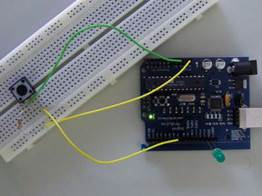Button1

int ledPin = 13; // choose the pin for the LED

int inPin = 2; // choose the input pin (for a pushbutton)

int val = 0; // variable for reading the pin status

void setup() {

pinMode(ledPin, OUTPUT); // declare LED as output

pinMode(inPin, INPUT); // declare pushbutton as input

}

void loop(){

if (val == HIGH) { // check if the input is HIGH (button released)

digitalWrite(ledPin, LOW); // turn LED OFF

} else {

digitalWrite(ledPin, HIGH); // turn LED ON

}

}

Debounce

This example demonstrates the use of a pushbutton as a switch: each time you press the button, the LED (or whatever) is turned on (if it's off) or off (if on). It also debounces the input, without which pressing the button once would appear to the

code as multiple presses. Makes use of the millis() function to keep track of the time when the button is pressed.

Circuit

A push-button on pin 7 and an LED on pin 13.

Code

int inPin = 7; // the number of the input pin

int outPin = 13; // the number of the output pin

int state = HIGH; // the current state of the output pin

int previous = LOW; // the previous reading from the input pin

// the follow variables are long's because the time, measured in miliseconds,

// will quickly become a bigger number than can be stored in an int.

long time = 0; // the last time the output pin was toggled

long debounce = 200; // the debounce time, increase if the output flickers

void setup()

{

pinMode(inPin, INPUT);

pinMode(outPin, OUTPUT);

}

void loop()

{

// if we just pressed the button (i.e. the input went from LOW to HIGH),

// and we've waited long enough since the last press to ignore any noise...

if (reading == HIGH && previous == LOW && millis() - time > debounce) {

// ... invert the output

if (state == HIGH)

state = LOW;

else

state = HIGH;

// ... and remember when the last button press was

time = millis();

}

digitalWrite(outPin, state);

}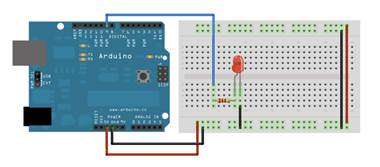/*

This example shows how to fade an LED on pin 9

using the analogWrite() function.

This example code is in the public domain.

*/

int led = 9;           // the pin that the LED is attached to

int brightness = 0;    // how bright the LED is

int fadeAmount = 5;    // how many points to fade the LED by

// the setup routine runs once when you press reset:

void setup()  {

// declare pin 9 to be an output:

pinMode(led, OUTPUT);

}

// the loop routine runs over and over again forever:

void loop()  {

// set the brightness of pin 9:

analogWrite(led, brightness);

// change the brightness for next time through the loop:

// reverse the direction of the fading at the ends of the fade:

if (brightness == 0 || brightness == 255) {

}

// wait for 30 milliseconds to see the dimming effect

delay(30);

}

Loop

We also call this example "Knight Rider" in memory to a TV-series from the 80's where the famous David Hasselhoff had an

AI machine driving his Pontiac. The car had been augmented with plenty of LEDs in all possible sizes performing flashy

effects.

Thus we decided that in order to learn more about sequential programming and good programming techniques for the I/O

board, it would be interesting to use the Knight Rider as a metaphor.

This example makes use of 6 LEDs connected to the pins 2 - 7 on the board using 220 Ohm resistors. The first code example

will make the LEDs blink in a sequence, one by one using only digitalWrite(pinNum,HIGH/LOW) and delay(time). The

second example shows how to use a for(;;) construction to perform the very same thing, but in fewer lines. The third and

last example concentrates in the visual effect of turning the LEDs on/off in a more softer way.

Circuit

Code

int timer = 100; // The higher the number, the slower the timing.

int pins[] = { 2, 3, 4, 5, 6, 7 }; // an array of pin numbers

int num_pins = 6; // the number of pins (i.e. the length of the array)

void setup()

{

int i;

for (i = 0; i < num pins; i++) // the array elements are numbered from 0 to num pins - 1

pinMode(pins[i], OUTPUT); // set each pin as an output

}

void loop()

{

int i;

for (i = 0; i < num_pins; i++) { // loop through each pin...

digitalWrite(pins[i], HIGH); // turning it on,

delay(timer); // pausing,

digitalWrite(pins[i], LOW); // and turning it off.

}

for (i = num_pins - 1; i >= 0; i--) {

digitalWrite(pins[i], HIGH);

delay(timer);

digitalWrite(pins[i], LOW);

}

}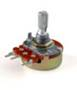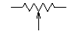Um potenciómetro (português europeu) é um componente eletrônico que possui resistência elétrica ajustável. Geralmente, é um resistor de três terminais onde a conexão central é deslizante e manipulável. Se todos os três terminais são usados, ele atua como um divisor de tensão

Exer Pot1

Exer Pot1 com potenciómetro saída de dados

Examples > 01. Basics > ReadAnalogVoltage

Um potenciômetro é um botão simples que fornece uma resistência variável, que se pode ler na placa Arduino como um valor análogo.

Neste exemplo, o valor controla a taxa em que um LED pisca.

Conectamos três fios à placa Arduino.

(Power – GND) - O primeiro vai para a terra (GND) de um dos pinos externos do potenciômetro;

(Power – 5v) o segundo vai de 5 volts para o outro pino exterior do potenciómetro.

3º (Analog in – A2) O terceiro vai de entrada analógica2 ao pino do meio do potenciômetro.

4º (Tools – Serial Monitor)

NOTA: Atenção à entrada da porta analógica

Exer Pot2

(Power – GND) - O primeiro vai para a terra (GND) de um dos pinos externos do potenciômetro;

(Power – 5v) o segundo vai de 5 volts para o outro pino exterior do potenciómetro.

3º (Analog in – A2) O terceiro vai de entrada analógica 2 ao pino do meio do potenciômetro.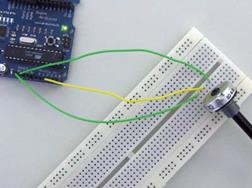Exer Pot3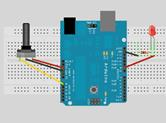/* POT to LED test -> by Owen Mundy March 11, 2010

—————————————————————*/

int potPin = 0;    // Analog input pin that the potentiometer is attached to

int potValue = 0;  // value read from the pot

int led = 9;      // PWM pin that the LED is on.  n.b. PWM 0 is on digital pin 9

void setup() {

// initialize serial communications at 9600 bps:

Serial.begin(9600);

// declare the led pin as an output:

pinMode(led, OUTPUT);

}

void loop() {

analogWrite(led, potValue/4);  // PWM the LED with the pot value (divided by 4 to fit in a byte)

Serial.println("hello");      // print the pot value back to the debugger pane

delay(10);                     // wait 10 milliseconds before the next loop

}

A potentiometer is a simple knob that provides a variable resistance, which we can read into the Arduino board as an analog value. In this example, that value controls the rate at which an LED blinks. We connect three wires to the Arduino board. The first goes to ground from one of the outer pins of the potentiometer. The second goes from 5 volts to the other outer pin of the potentiometer. The third goes from analog input 2 to the middle pin of the potentiometer. By turning the shaft of the potentiometer, we change the amount of resistence on either side of the wiper which is connected

to the center pin of the potentiometer. This changes the relative "closeness" of that pin to 5 volts and ground, giving us a different analog input. When the shaft is turned all the way in one direction, there are 0 volts going to the pin, and we read 0. When the shaft is turned all the way in the other direction, there are 5 volts going to the pin and we read 1023. In between, analogRead() returns a number between 0 and 1023 that is proportional to the amount of voltage being applied to the pin.

Code

/*

* by DojoDave <http://www.0j0.org>

*

* Turns on and off a light emitting diode(LED) connected to digital

* pin 13. The amount of time the LED will be on and off depends on

* the value obtained by analogRead(). In the easiest case we connect

* a potentiometer to analog pin 2.

*/

int potPin = 2; // select the input pin for the potentiometer

int ledPin = 13; // select the pin for the LED

int val = 0; // variable to store the value coming from the sensor

void setup() {

pinMode(ledPin, OUTPUT); // declare the ledPin as an OUTPUT

}

void loop() {

digitalWrite(ledPin, HIGH); // turn the ledPin on

delay(val); // stop the program for some time

digitalWrite(ledPin, LOW); // turn the ledPin off

delay(val); // stop the program for some time

}

Demonstrates the use of analog output (PWM) to fade an LED.

Circuit

An LED connected to digital pin 9.

Code

int value = 0; // variable to keep the actual value

int ledpin = 9; // light connected to digital pin 9

void setup()

{

// nothing for setup

}

void loop()

{

for(value = 0 ; value <= 255; value+=5) // fade in (from min to max)

{

analogWrite(ledpin, value); // sets the value (range from 0 to 255)

delay(30); // waits for 30 milli seconds to see the dimming effect

}

for(value = 255; value >=0; value-=5) // fade out (from max to min)

{

analogWrite(ledpin, value);

delay(30);

}

}

Pag 20

LOOP

VOLTAR

15

Loop

Acender de leds sequencial -  este exemplo faz com que 6 leds conectados aos pinos 2 a 7

6 led

6 Resistencias de 220

Recurso de programação digitalWrite(pinNum,HIGH/LOW) and delay(time

We also call this example "Knight Rider" in memory to a TV-series from the 80's where the famous David Hasselhoff had an AI machine driving his Pontiac. The car had been augmented with plenty of LEDs in all possible sizes performing flashy effects. Thus we decided that in order to learn more about sequential programming and good programming techniques for the I/O board, it would be interesting to use the Knight Rider as a metaphor. This example makes use of 6 LEDs connected to the pins 2 - 7 on the board using 220 Ohm resistors. The first code example will make the LEDs blink in a sequence, one by one using only digitalWrite(pinNum,HIGH/LOW) and delay(time). The second example shows how to use a for(;;) construction to perform the very same thing, but in fewer lines. The third and last example concentrates in the visual effect of turning the LEDs on/off in a more softer way.

Code

int timer = 100; // inicializar o timer quanto maior o nº mais tempo demora)The higher the number, the slower the timing.

int pins[] = { 2, 3, 4, 5, 6, 7 }; // definição dos pins de saída - an array of pin numbers

int num_pins = 6; // difine o nº de pins - the number of pins (i.e. the length of the array)

void setup()

{

int i;

for (i = 0; i < num pins; i++) // Para i =0 até i nmpins i++ incrementa 1) the array elements are numbered from 0 to num pins - 1

pinMode(pins[i], OUTPUT); // define os pins para otput set each pin as an output

}

void loop()

{

int i;

for (i = 0; i < num_pins; i++) { // loop through each pin...

digitalWrite(pins[i], HIGH); // turning it on,

delay(timer); // pausing,

digitalWrite(pins[i], LOW); // and turning it off.

}

for (i = num_pins - 1; i >= 0; i--) {

digitalWrite(pins[i], HIGH);

delay(timer);

digitalWrite(pins[i], LOW);

}

}

VOLTAR

Imprime os caracteres ascii

Não é necessário circuito, apenas software + ferramenta serial print do arduino

Demonstrates the advanced serial printing functions by generating a table of characters and their ASCII values in decimal,

Circuit

None, but the Arduino has to be connected to the computer.

Code

// ASCII Table

// by Nicholas Zambetti <http://www.zambetti.com>

void setup()

{

Serial.begin(9600);

// prints title with ending line break

Serial.println("ASCII Table ~ Character Map");

// wait for the long string to be sent

delay(100);

}

int number = 33; // first visible character '!' is #33

void loop()

{

Serial.print(number, BYTE); // prints value unaltered, first will be '!'

Serial.print(", dec: ");

Serial.print(number); // prints value as string in decimal (base 10)

// Serial.print(number, DEC); // this also works

Serial.print(", hex: ");

Serial.print(number, HEX); // prints value as string in hexadecimal (base 16)

Serial.print(", oct: ");

Serial.print(number, OCT); // prints value as string in octal (base 8)

Serial.print(", bin: ");

Serial.println(number, BIN); // prints value as string in binary (base 2)

// also prints ending line break

// if printed last visible character '~' #126 ...

if(number == 126) {

// loop forever

while(true) {

continue;

}

}

number++; // to the next character

delay(100); // allow some time for the Serial data to be sent

}

Output

ASCII Table ~ Character Map

!, dec: 33, hex: 21, oct: 41, bin: 100001

", dec: 34, hex: 22, oct: 42, bin: 100010

#, dec: 35, hex: 23, oct: 43, bin: 100011

\$, dec: 36, hex: 24, oct: 44, bin: 100100

%, dec: 37, hex: 25, oct: 45, bin: 100101

&, dec: 38, hex: 26, oct: 46, bin: 100110

', dec: 39, hex: 27, oct: 47, bin: 100111

(, dec: 40, hex: 28, oct: 50, bin: 101000

VOLTAR

Examples > Communication

Dimmer

Enviar dados do computador para o arduino – neste caso controlar a intensidade de luz de um led

Demonstrates the sending data from the computer to the Arduino board, in this case to control the brightness of an LED. The data is sent in individual bytes, each of which ranges from 0 to 255. Arduino reads these bytes and uses them to set the brightness of the LED.

Circuit

An LED connected to pin 9 (with appropriate resistor).

Code

int ledPin = 9;

void setup()

{

// begin the serial communication

Serial.begin(9600);

pinMode(ledPin, OUTPUT);

}

void loop()

{

byte val;

// check if data has been sent from the computer

if (Serial.available()) {

// read the most recent byte (which will be from 0 to 255)

// set the brightness of the LED

analogWrite(ledPin, val);

}

}

Processing Code

// Dimmer - sends bytes over a serial port

// by David A. Mellis

import processing.serial.*;

Serial port;

void setup()

{

size(256, 150);

println("Available serial ports:");

println(Serial.list());

// Uses the first port in this list (number 0). Change this to

// select the port corresponding to your Arduino board. The last

// parameter (e.g. 9600) is the speed of the communication. It

// has to correspond to the value passed to Serial.begin() in your

// Arduino sketch.

port = new Serial(this, Serial.list(), 9600);

// If you know the name of the port used by the Arduino board, you

// can specify it directly like this.

//port = new Serial(this, "COM1", 9600);

}

void draw()

{

// draw a gradient from black to white

for (int i = 0; i < 256; i++) {

stroke(i);

line(i, 0, i, 150);

}

// write the current X-position of the mouse to the serial port as

// a single byte

port.write(mouseX);

VOLTAR

Examples > Communication

Graph

A simple example of communication from the Arduino board to the computer: the value of an analog input is printed. We call this "serial" communication because the connection appears to both the Arduino and the computer as an old-fashioned serial port, even though it may actually use a USB cable.

You can use the Arduino serial monitor to view the sent data, or it can be read by Processing (see code below), Flash, PD, Max/MSP, etc.

Circuit

Code

void setup()

{

Serial.begin(9600);

}

void loop()

{

delay(20);

}

Processing Code

// Graph

// by David A. Mellis

//

// Demonstrates reading data from the Arduino board by graphing the

//

// by <a href="http://itp.jtnimoy.com">Josh Nimoy</a>.

import processing.serial.*;

Serial port;

String buff = "";

int NEWLINE = 10;

// Store the last 64 values received so we can graph them.

int[] values = new int;

void setup()

{

size(512, 256);

println("Available serial ports:");

println(Serial.list());

// Uses the first port in this list (number 0). Change this to

// select the port corresponding to your Arduino board. The last

// parameter (e.g. 9600) is the speed of the communication. It

// has to correspond to the value passed to Serial.begin() in your

// Arduino sketch.

port = new Serial(this, Serial.list(), 9600);

// If you know the name of the port used by the Arduino board, you

// can specify it directly like this.

//port = new Serial(this, "COM1", 9600);

}

void draw()

{

background(53);

stroke(255);

// Graph the stored values by drawing a lines between them.

for (int i = 0; i < 63; i++)

line(i * 8, 255 - values[i], (i + 1) * 8, 255 - values[i + 1]);

while (port.available() > 0)

}

void serialEvent(int serial)

{

if (serial != NEWLINE) {

// Store all the characters on the line.

buff += char(serial);

} else {

// The end of each line is marked by two characters, a carriage

// return and a newline. We're here because we've gotten a newline,

// but we still need to strip off the carriage return.

buff = buff.substring(0, buff.length()-1);

// Parse the String into an integer. We divide by 4 because

// analog inputs go from 0 to 1023 while colors in Processing

// only go from 0 to 255.

int val = Integer.parseInt(buff)/4;

// Clear the value of "buff"

buff = "";

// Shift over the existing values to make room for the new one.

for (int i = 0; i < 63; i++)

values[i] = values[i + 1];

values = val;

}

}

VOLTAR

Examples > Communication

Physical Pixel

Do pc para o arduino – neste caso acende o led quando carregamos em H e apaga em L

An example of using the Arduino board to receive data from the computer. In this case, the Arduino boards turns on an LED when it receives the character 'H', and turns off the LED when it receives the character 'L'. The data can be sent from the Arduino serial monitor, or another program like Processing (see code below), Flash (via a serial-net proxy), PD, or Max/MSP.

Circuit

An LED on pin 13.

Code

int outputPin = 13;

int val;

void setup()

{

Serial.begin(9600);

pinMode(outputPin, OUTPUT);

}

void loop()

{

if (Serial.available()) {

if (val == 'H') {

digitalWrite(outputPin, HIGH);

}

if (val == 'L') {

digitalWrite(outputPin, LOW);

}

}

}

Processing Code

// mouseover serial

// by BARRAGAN <http://people.interaction-ivrea.it/h.barragan>

// Demonstrates how to send data to the Arduino I/O board, in order to

// turn ON a light if the mouse is over a rectangle and turn it off

// if the mouse is not.

// created 13 May 2004

import processing.serial.*;

Serial port;

void setup()

{

size(200, 200);

noStroke();

frameRate(10);

// List all the available serial ports in the output pane.

// You will need to choose the port that the Arduino board is

// connected to from this list. The first port in the list is

// port #0 and the third port in the list is port #2.

println(Serial.list());

// Open the port that the Arduino board is connected to (in this case #0)

// Make sure to open the port at the same speed Arduino is using (9600bps)

port = new Serial(this, Serial.list(), 9600);

}

// function to test if mouse is over square

boolean mouseOverRect()

{

return ((mouseX >= 50)&&(mouseX <= 150)&&(mouseY >= 50)&(mouseY <= 150));

}

void draw()

{

background(#222222);

if(mouseOverRect()) // if mouse is over square

{

fill(#BBBBB0); // change color

port.write('H'); // send an 'H' to indicate mouse is over square

} else {

fill(#666660); // change color

port.write('L'); // send an 'L' otherwise

}

rect(50, 50, 100, 100); // draw square

}

VOLTAR

Virtual Color Mixer

Do arduino para o PC – neste caso informação de 3 potenciómetros

Demonstrates one technique for sending multiple values from the Arduino board to the computer. In this case, the readings

from three potentiometers are used to set the red, green, and blue components of the background color of a Processing

sketch.

Circuit

Code

int redPin = 0;

int greenPin = 1;

int bluePin = 2;

void setup()

{

Serial.begin(9600);

}

void loop()

{

Serial.print("R");

Serial.print("G");

Serial.print("B");

delay(100);

}

Processing Code

/**

* Color Mixer

* by David A. Mellis

*

* Created 2 December 2006

*

* by <a href="http://itp.jtnimoy.com">Josh Nimoy</a>.

*

* Created 8 February 2003

* Updated 2 April 2005

*/

import processing.serial.*;

String buff = "";

int rval = 0, gval = 0, bval = 0;

int NEWLINE = 10;

Serial port;

void setup()

{

size(200, 200);

// Print a list in case COM1 doesn't work out

println("Available serial ports:");

println(Serial.list());

//port = new Serial(this, "COM1", 9600);

// Uses the first available port

port = new Serial(this, Serial.list(), 9600);

}

void draw()

{

while (port.available() > 0) {

}

background(rval, gval, bval);

}

void serialEvent(int serial)

{

// If the variable "serial" is not equal to the value for

// a new line, add the value to the variable "buff". If the

// value "serial" is equal to the value for a new line,

// save the value of the buffer into the variable "val".

if(serial != NEWLINE) {

buff += char(serial);

} else {

// The first character tells us which color this value is for

char c = buff.charAt(0);

// Remove it from the string

buff = buff.substring(1);

// Discard the carriage return at the end of the buffer

buff = buff.substring(0, buff.length()-1);

// Parse the String into an integer

if (c == 'R')

rval = Integer.parseInt(buff);

else if (c == 'G')

gval = Integer.parseInt(buff);

else if (c == 'B')

bval = Integer.parseInt(buff);

// Clear the value of "buff"

buff = "";

}

}

VOLTAR

Read Two Switches With One I/O Pin

There are handy 20K pullup resistors (resistors connected internally between Arduino I/O pins and VCC - +5 volts in the

Arduino's case) built into the Atmega chip upon which Freeduino's are based. They are accessible from software by using the

digitalWrite() function, when the pin is set to an input.

This sketch exploits the pullup resistors under software control. The idea is that an external 200K resistor to ground will

cause an input pin to report LOW when the internal (20K) pullup resistor is turned off. When the internal pullup resistor is

turned on however, it will overwhelm the external 200K resistor and the pin will report HIGH.

One downside of the scheme (there always has to be a downside doesn't there?) is that one can't tell if both buttons are

pushed at the same time. In this case the scheme just reports that sw2 is pushed. The job of the 10K series resistor,

incidentally, is to prevent a short circuit if a pesky user pushes both buttons at once. It can be omitted on a center-off slide

or toggle switch where the states are mutually exclusive.

/*

* Read two pushbutton switches or one center-off toggle switch with one Arduino pin

* From an idea in EDN (Electronic Design News)

*

* Exploits the pullup resistors available on each I/O and analog pin

* The idea is that the 200K resistor to ground will cause the input pin to report LOW when the

* (20K) pullup resistor is turned off, but when the pullup resistor is turned on,

* it will overwhelm the 200K resistor and the pin will report HIGH.

*

* Schematic Diagram ( can't belive I drew this funky ascii schematic )

*

*

* +5 V

* |

* \

* /

* \ 10K

* /

* \

* |

* / switch 1 or 1/2 of center-off toggle or slide switch

* /

* |

* digital pin ________+_____________/\/\/\____________ ground

* |

* | 200K to 1M (not critical)

* /

* / switch 2 or 1/2 of center-off toggle or slide switch

* |

* |

* _____

* ___ ground

* _

*

*/

#define swPin 2 // pin for input - note: no semicolon after #define

int stateA, stateB; // variables to store pin states

int sw1, sw2; // variables to represent switch states

void setup()

{

Serial.begin(9600);

}

void loop()

{

digitalWrite(swPin, LOW); // make sure the puillup resistors are off

digitalWrite(swPin, HIGH); // turn on the puillup resistors

if ( stateA == 1 && stateB == 1 ){ // both states HIGH - switch 1 must be pushed

sw1 = 1;

sw2 = 0;

}

else if ( stateA == 0 && stateB == 0 ){ // both states LOW - switch 2 must be pushed

sw1 = 0;

sw2 = 1;

}

else{ // stateA HIGH and stateB LOW

sw1 = 0; // no switches pushed - or center-off toggle in middle

position

sw2 = 0;

}

Serial.print(sw1);

Serial.print(" "); // pad some spaces to format print output

Serial.println(sw2);

delay(100);

}

 Controlar Arduino via Internet How to remotely enable/disable a LED on your Arduino using PHP and Processing Ótimo exemplo Dúvidas sobre processing consultar https://www.youtube.com/watch?v=g0pSfyXOXj8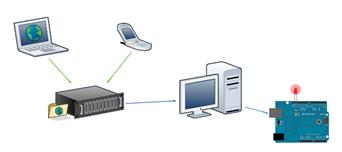Serve para estes dois   Estes 2 complementam-se Testado e aprovado Tutorial 06 for Arduino: Serial Communication and Processing Ferramentas Site para download da ferramenta